# Plotting VWP Data Processed with VWPre

## Plotting the data

It’s often desirable to visualize the proportion (or empirical logit) data, either as a grand average or by condition. In some cases it is even necessary to visualize the trend in the data over a continuous predictor. So, the functions plot_avg and plot_avg_contour provide straightforward plotting options for such cases. These functions internally calculate the average(s) and plot the results. The plotting is powered by ggplot2, so further customization (plot titles, custom themes, etc.) is still possible. For more information about ggplot2, please refer to its reference manual and extensive documentation.

### Averaged data

Using the function plot_avg, it is possible to plot the overall average of the data by interest area. The parameter type specifies which type of plot to create: proportion or empirical logit. In IAColumns, list the interest area proportion columns (here we have used the default names) along with desired labels.

plot_avg(data = dat, type = "proportion", xlim = c(0, 1000),
IAColumns = c(IA_1_P = "Target", IA_2_P = "Rhyme", IA_3_P = "OnsetComp",
IA_4_P = "Distractor"),
Condition1 = NULL, Condition2 = NULL, Cond1Labels = NA, Cond2Labels = NA,
ErrorBar = TRUE, VWPreTheme = TRUE)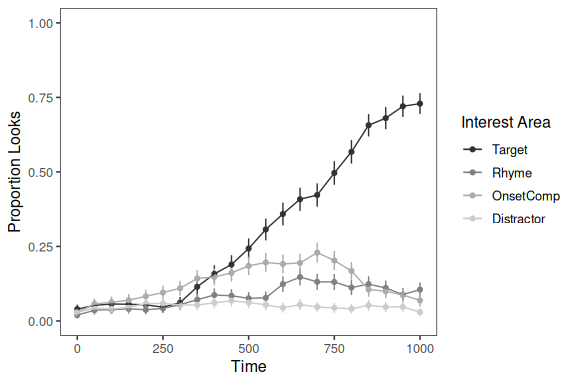To add a title to the plot, simply add the title function from ggplot2.

plot_avg(data = dat, type = "proportion", xlim = c(0, 1000),
IAColumns = c(IA_1_P = "Target", IA_2_P = "Rhyme", IA_3_P = "OnsetComp",
IA_4_P = "Distractor"),
Condition1 = NULL, Condition2 = NULL, Cond1Labels = NA, Cond2Labels = NA,
ErrorBar = TRUE, VWPreTheme = TRUE) + ggtitle("Averaged Data")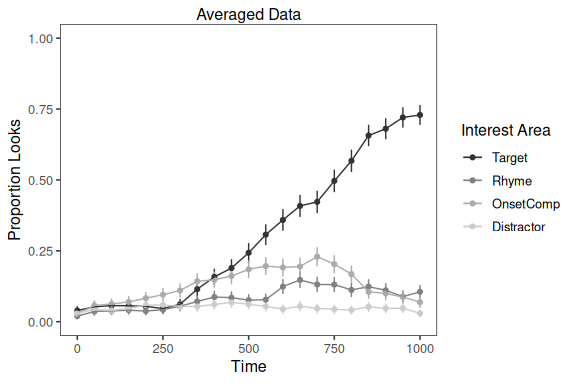To customize the appearance of a plot (e.g., font, size, color, margins, etc.), the VWPreTheme parameter can be set to FALSE, which reverts to the default theming in gglpot2. In this way, the user can apply a custom theme to the plot. Detailed information about creating themes can be found at ggplot2 Theme Vignette. For the purpose of illustration, the default ggplot2 theme has been applied, with the axis text elements increased in size.

plot_avg(data = dat, type = "proportion", xlim = c(0, 1000),
IAColumns = c(IA_1_P = "Target", IA_2_P = "Rhyme", IA_3_P = "OnsetComp",
IA_4_P = "Distractor"),
Condition1 = NULL, Condition2 = NULL, Cond1Labels = NA, Cond2Labels = NA,
ErrorBar = TRUE, VWPreTheme = FALSE) + theme(axis.text = element_text(size = 15))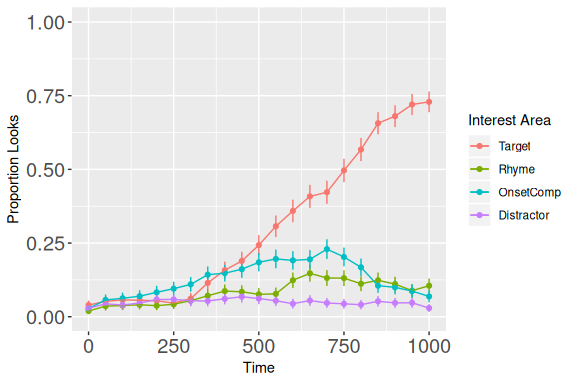### Conditional averages

The function plot_avg can also be used to plot averages for different conditions, based on a factor variable in the data. If the current factor level labels are not suitable for plotting, specify new labels using a list in Cond1Labels.

Specifying Condition1 will stack the plots.

plot_avg(data = dat, type = "proportion", xlim = c(0, 1000),
IAColumns = c(IA_1_P = "Target", IA_2_P = "Rhyme", IA_3_P = "OnsetComp",
IA_4_P = "Distractor"), Condition1 = "talker",
Condition2 = NULL, Cond1Labels = c(CH1 = "Chinese 1", CH10 = "Chinese 3",
CH9 = "Chinese 2", EN3 = "English 1"),
Cond2Labels = NA, ErrorBar = TRUE, VWPreTheme = TRUE)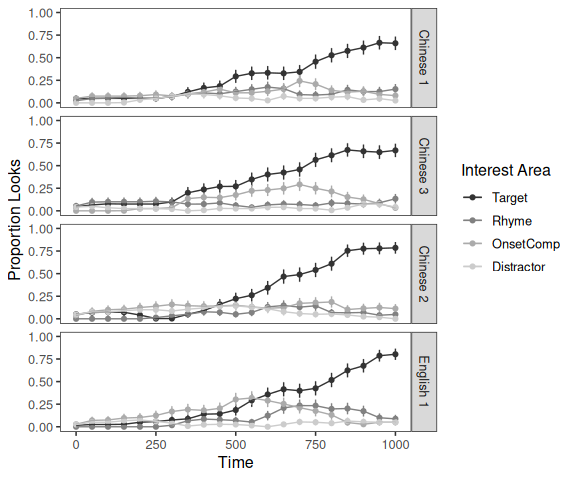Alternatively, specifying just Condition2 will plot the same information, but align it horizontally.

plot_avg(data = dat, type = "proportion", xlim = c(0, 1000),
IAColumns = c(IA_1_P = "Target", IA_2_P = "Rhyme", IA_3_P = "OnsetComp",
IA_4_P = "Distractor"), Condition1 = NULL,
Condition2 = "talker", Cond1Labels = NA, Cond2Labels = c(CH1 = "Chinese 1",
CH10 = "Chinese 3",
CH9 = "Chinese 2",
EN3 = "English 1"),
ErrorBar = TRUE, VWPreTheme = TRUE)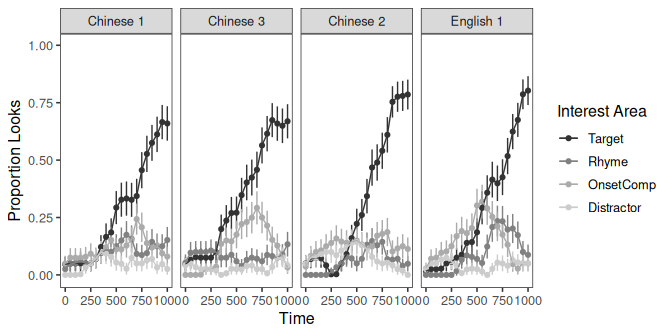For a 2x2 design, it is possible to specify both conditions. This will create a grid-style plot.

plot_avg(data = dat, type = "proportion", xlim = c(0, 1000),
IAColumns = c(IA_1_P = "Target", IA_2_P = "Rhyme", IA_3_P = "OnsetComp",
IA_4_P = "Distractor"), Condition1 = "talker",
Condition2 = "Exp", Cond1Labels = c(CH1 = "Chinese 1", CH10 = "Chinese 3",
CH9 = "Chinese 2", EN3 = "English 1"),
Cond2Labels = c(High = "High Exp", Low = "Low Exp"), ErrorBar = TRUE,
VWPreTheme = TRUE)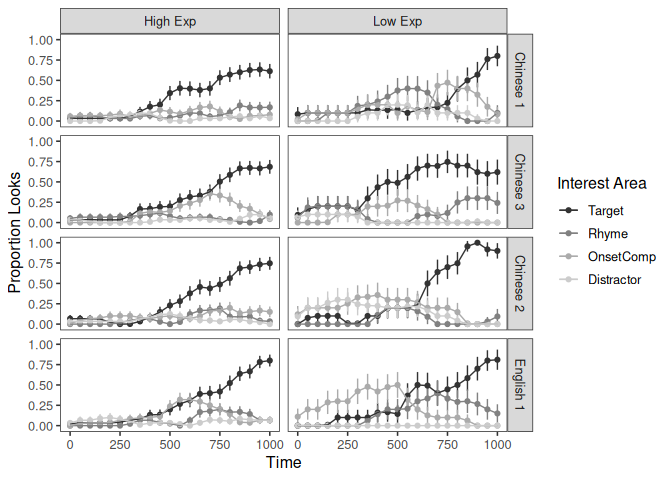### Error bars and Error bands

Error around the means can be plotted in two ways, either as error bars or as error bands. Note that with the included theme, the colors and shapes change for error bands to maximize readablity.

gridExtra::grid.arrange(

plot_avg(data = dat, type = "proportion", xlim = c(0, 1000),
IAColumns = c(IA_1_P = "Target", IA_2_P = "Rhyme", IA_3_P = "OnsetComp",
IA_4_P = "Distractor"),
Condition1 = NULL, Condition2 = NULL, Cond1Labels = NA, Cond2Labels = NA,
ErrorBar = TRUE, VWPreTheme = TRUE),

plot_avg(data = dat, type = "proportion", xlim = c(0, 1000),
IAColumns = c(IA_1_P = "Target", IA_2_P = "Rhyme", IA_3_P = "OnsetComp",
IA_4_P = "Distractor"),
Condition1 = NULL, Condition2 = NULL, Cond1Labels = NA, Cond2Labels = NA,
ErrorBar = FALSE, ErrorBand = TRUE, VWPreTheme = TRUE),

nrow=2, ncol=1)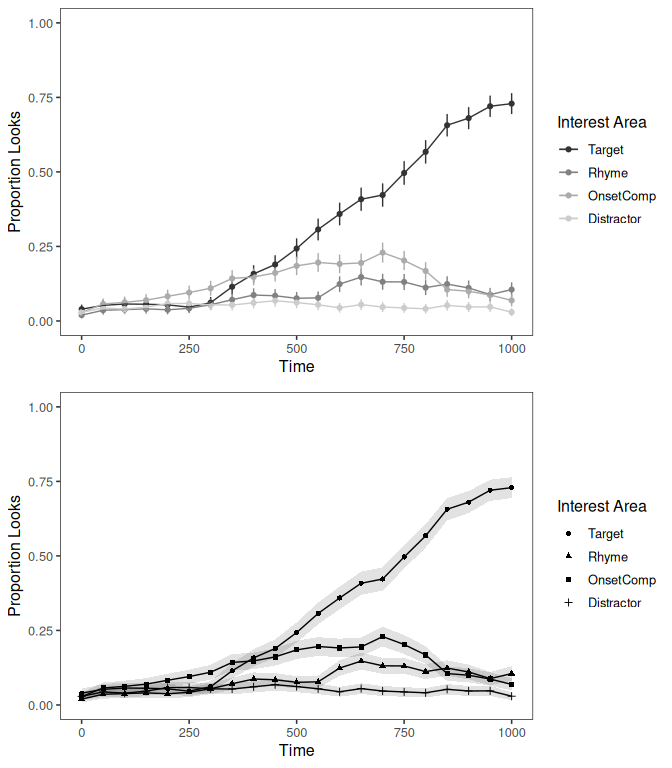### Confidence intervals (pointwise/simultaneous)

Rather than having standard error plotted, it is possible to have confidence intervals added to the graph. Confidence intervals can be plotted either as bars or bands and controlled using ErrorType and ConfLev. ErrorType should be set to “CI” for confidence intervals, and ConfLev set to the desired levels of confidence (by default 95). An important option is the type of desired interval, either pointwise or simultaneous, using CItype. Pointwise use the confidence level provided for each interval plotted. Simultaneous are, on the other hand, corrected for multiple intervals using the Bonferroni method. Underlyingly this correction is done using the number of data points along the x-axis (Time).

gridExtra::grid.arrange(

plot_avg(data = dat, type = "proportion", xlim = c(0, 1000),
IAColumns = c(IA_1_P = "Target", IA_2_P = "Rhyme", IA_3_P = "OnsetComp",
IA_4_P = "Distractor"),
Condition1 = NULL, Condition2 = NULL, Cond1Labels = NA, Cond2Labels = NA,
ErrorBar = FALSE, ErrorBand = TRUE, ErrorType = "CI", ConfLev = 95,
CItype = "pointwise", VWPreTheme = TRUE),

plot_avg(data = dat, type = "proportion", xlim = c(0, 1000),
IAColumns = c(IA_1_P = "Target", IA_2_P = "Rhyme", IA_3_P = "OnsetComp",
IA_4_P = "Distractor"),
Condition1 = NULL, Condition2 = NULL, Cond1Labels = NA, Cond2Labels = NA,
ErrorBar = FALSE, ErrorBand = TRUE, ErrorType = "CI", ConfLev = 95,
CItype = "simultaneous", VWPreTheme = TRUE),

nrow=2, ncol=1)
## Grand average calculated using Event means.
## Plot created with pointwise confindence intervals (set to 95%).
## Grand average calculated using Event means.
## Plot created with simultaneous confindence intervals (adjusted to 99.88% using the Bonferroni method).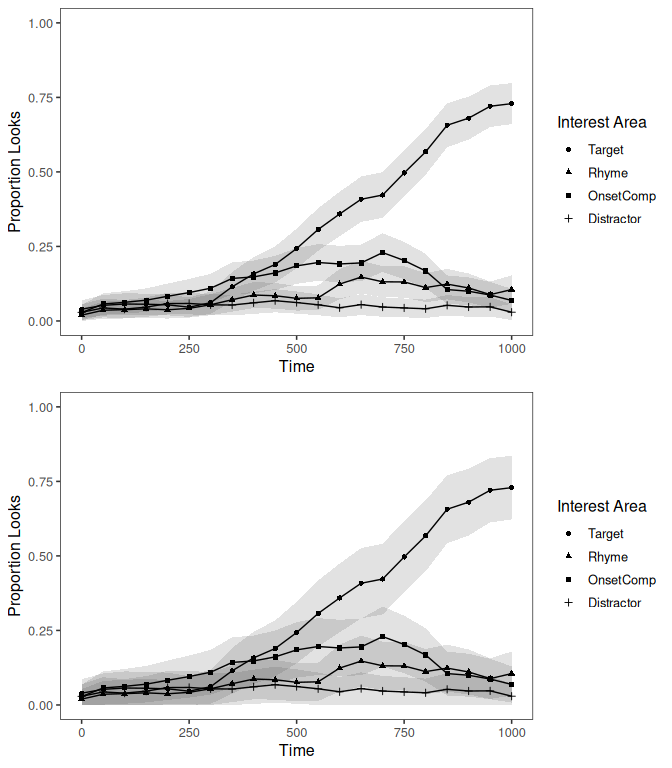### Single interest area by condition

When plotting a single interest area by condition, it is very typical to plot the conditional averages in a single frame (rather than in separate facets) to facilitate visual comparison. If only one interest area is given, the function will output a figure in which the conditional curves are visualized in a single frame.

plot_avg(data = dat, type = "proportion", xlim = c(0, 1000),
IAColumns = c(IA_1_P = "Target"), Condition1 = "talker",
Condition2 = NULL, Cond1Labels = c(CH1 = "Chinese 1", CH10 = "Chinese 3",
CH9 = "Chinese 2", EN3 = "English 1"),
Cond2Labels = NA, ErrorBar = TRUE, VWPreTheme = TRUE)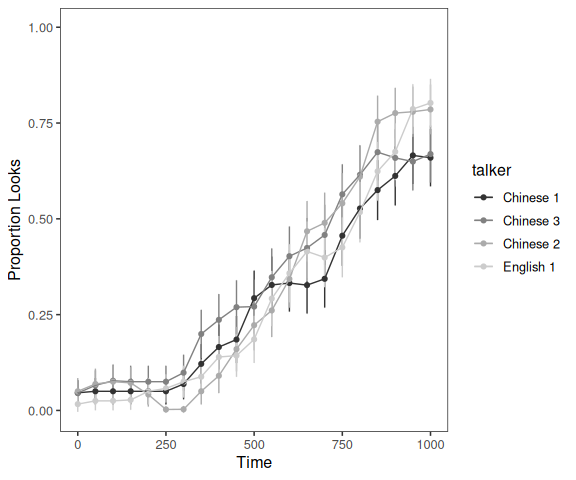### Calculation of averages - Mean vs. Grand mean

By default, the plotting function calculates the overall mean in the data set for a given interest area by time. However, by changing the input to the parameter Averaging, it is possible to supply a column name for which grand mean (mean of means) can be calculated (e.g., “Subject”). Note that for balanced data, the resultant mean will be the same, but the size of the error bars/bands will change. For example, given the small number of participants in this data set, the error bars become much wider.

plot_avg(data = dat, type = "proportion", xlim = c(0, 1000),
IAColumns = c(IA_1_P = "Target", IA_2_P = "Rhyme", IA_3_P = "OnsetComp",
IA_4_P = "Distractor"),
Condition1 = NULL, Condition2 = NULL, Cond1Labels = NA, Cond2Labels = NA,
Averaging = "Subject", ErrorBar = TRUE, ErrorType = "SE", VWPreTheme = TRUE)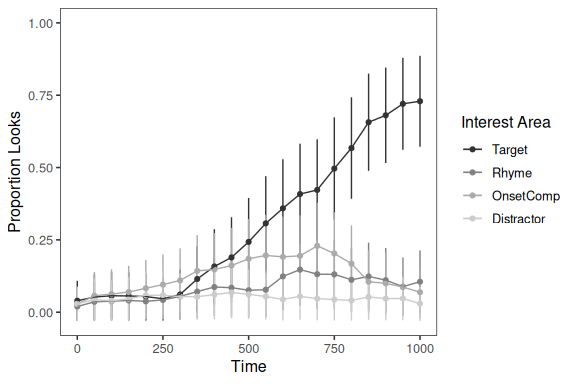### Difference plots (between looks to two interest areas)

The function plot_avg_diff can also be used to plot the average difference between looks to two interest areas. As with plot_avg up to two conditions can be supplied for conditional plotting.

gridExtra::grid.arrange(

plot_avg_diff(data = dat, type = "proportion", xlim = c(0, 1000),
DiffCols = c(IA_1_P = "Target", IA_2_P = "Rhyme"),
Condition1 = NULL, Condition2 = NULL, Cond1Labels = NA,
Cond2Labels = NA, ErrorBar = TRUE, VWPreTheme = TRUE),

plot_avg_diff(data = dat, type = "proportion", xlim = c(0, 1000),
DiffCols = c(IA_1_P = "Target", IA_2_P = "Rhyme"),
Condition1 = "talker", Condition2 = NULL, Cond1Labels = c(CH1 = "Chinese 1",
CH10 = "Chinese 3", CH9 = "Chinese 2", EN3 = "English 1"),
Cond2Labels = NA, ErrorBar = TRUE, VWPreTheme = TRUE),

nrow=2, ncol=1)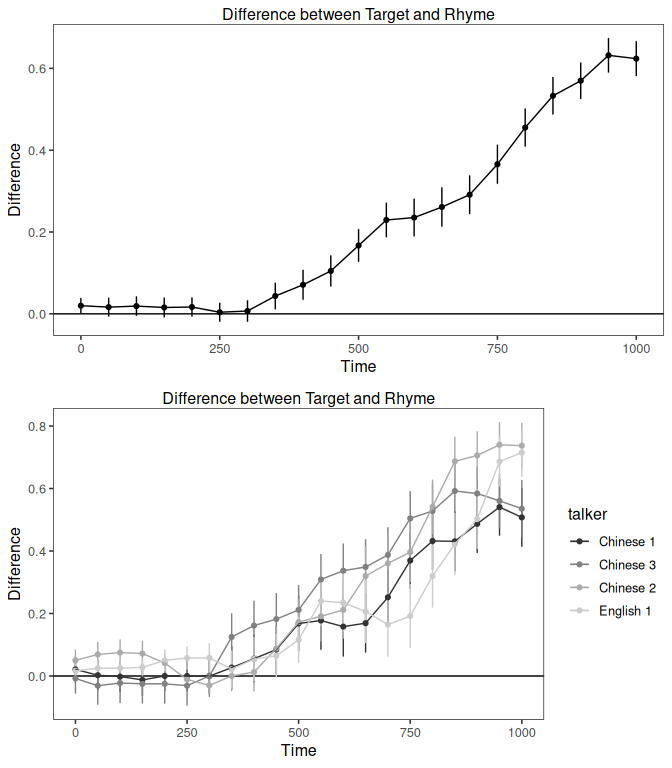Error bands, confidence intervals (both pointwise and simultaneous), and averaging work the same way as in the plot_avg function.

### Difference plots (between two conditions for looks to one interest area)

The function plot_avg_cdiff can also be used to plot the average difference between two conditions for looks to a single interest area.

plot_avg_cdiff(data = dat, type = "proportion", xlim = c(0, 1000),
IAColumn = "IA_1_P", Condition = list(talker = c("EN3", "CH1")),
CondLabels = c(CH1 = "Chinese 1", EN3 = "English 1"),
ErrorBar = TRUE, VWPreTheme = TRUE, Averaging = "Subject")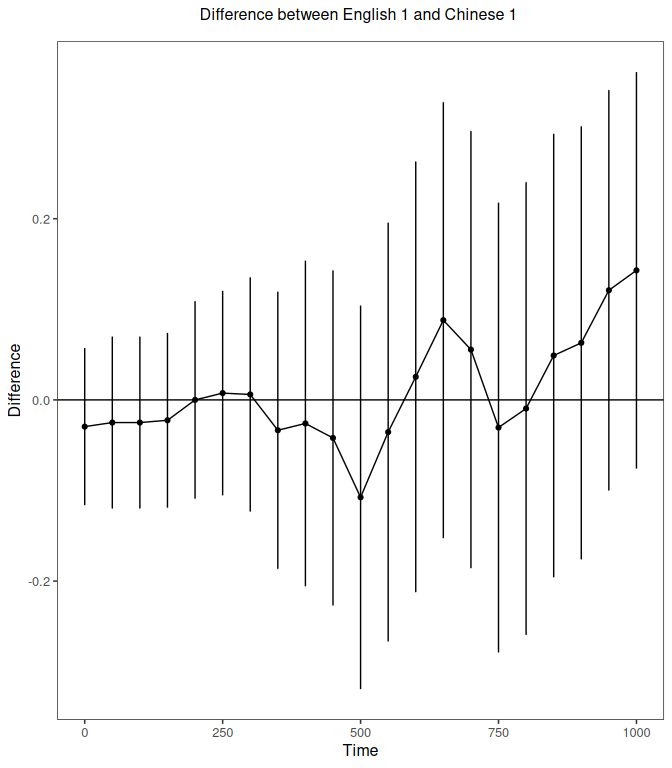Again, error bands and confidence intervals (both pointwise and simultaneous) work the same way as in the plot_avg function. With regard to averaging, please note that the function will check that both levels of the condition occur for each level of the column provided in Averaging (e.g., Subject or Item), as this is necessary to calculate the difference based on subject or item means. If this is not the case, the function will stop. If you would still like to produce the plot based on the difference between sample means of the levels, you can set Averaging = NULL.

### Conditional contour surface

In some cases, studies have not employed a factorial design; rather they aim to investigate continuous variables. Therefore, using the function plot_avg_contour it is also possible to create a contour plot representing the looks to one interest area as a surface over the continuous variable and Time. This function calculates the average time series at each value of the continuous variable and applies a smooth (utilizing the function gam in the package mgcv) over the surface. The function then plots the result as a contour plot. Here, the example plots looks to the target as a function of Rating and Time.

plot_avg_contour(data = dat, IA = "IA_1_P", type = "proportion", Var = "Rating",
VarLabel = "Accent Rating", xlim = c(0,1000), VWPreTheme = TRUE,
Colors = c("gray20", "gray90"))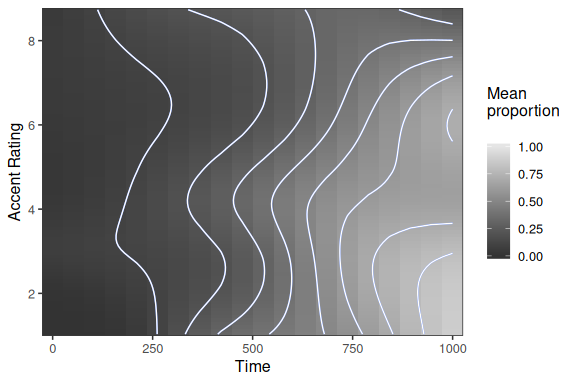It is possible to change the contour colors and add a title. ggplot2 accepts predefined palette colors, RGB, hexadecimal, among others.

plot_avg_contour(data = dat, IA = "IA_1_P", type = "proportion", Var = "Rating",
VarLabel = "Accent Rating", xlim = c(0,1000), VWPreTheme = TRUE,
Colors = c("red", "green")) + ggtitle("Looks to target")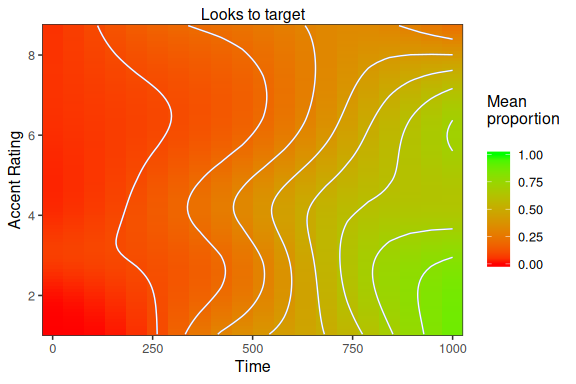Averaging works the same way as in the plot_avg function.

## Extracting plotting data

Because the plotting functions are based on ggplot2 it is possible to easily extract the data used to create the plots.
This is useful if you desire a highly custom plot, but would like to use the averages and error calculations produced by the plotting function.

To extract the data, first save the plot to an object in your workspace. This will create a list object containing the data.

plt <- plot_avg(data = dat, type = "proportion", xlim = c(0, 1000),
IAColumns = c(IA_1_P = "Target", IA_2_P = "Rhyme", IA_3_P = "OnsetComp",
IA_4_P = "Distractor"),
Condition1 = NULL, Condition2 = NULL, Cond1Labels = NA, Cond2Labels = NA,
ErrorBar = TRUE, VWPreTheme = TRUE) 

The data can then be accessed by extracting the dataframe from the list.

df <- plt\$data
IA Time mean n se ci error_lower error_upper
IA_1_P 0 0.039500 160 0.0153988 0.0467827 0.0241012 0.0548988
IA_1_P 50 0.052375 160 0.0176125 0.0535080 0.0347625 0.0699875
IA_1_P 100 0.056875 160 0.0183099 0.0556268 0.0385651 0.0751849
IA_1_P 150 0.056125 160 0.0181960 0.0552807 0.0379290 0.0743210
IA_1_P 200 0.054125 160 0.0178878 0.0543443 0.0362372 0.0720128
IA_1_P 250 0.046250 160 0.0166040 0.0504442 0.0296460 0.0628540

## Backtransformation of predicted values

The function make_pelogit_fnc creates a function that can be used to transform the empirical logit scale back to probability scale. The function must be supplied with the number of samples and the constant used in the original transformation. This is particularly handy for backtransforming model predicitions to probability scale.

model <- mgcv::bam(IA_1_ELogit ~ s(Time), data = dat)
pelogit <- make_pelogit_fnc(ObsPerBin=50, Constant=0.5)
df <- data.frame(Fitted = fitted(model), Backtransformed = pelogit(fitted(model)))

Here we see the backtransformed values.

Fitted Backtransformed
10 -3.5689822 0.0179602
11 -3.2079483 0.0296451
12 -2.7840408 0.0493566
13 -2.3298873 0.0804513
14 -1.8694714 0.1262750
15 -1.4087872 0.1903539
16 -0.9367525 0.2771879
17 -0.4368157 0.3903500
18 0.0958843 0.5244318
19 0.6413638 0.6581629
20 1.1553112 0.7656893

Here we see how the function can be included in the plotting functions of other packages.

par(mfrow=c(2,1))
itsadug::plot_smooth(model, view = "Time", transform = pelogit)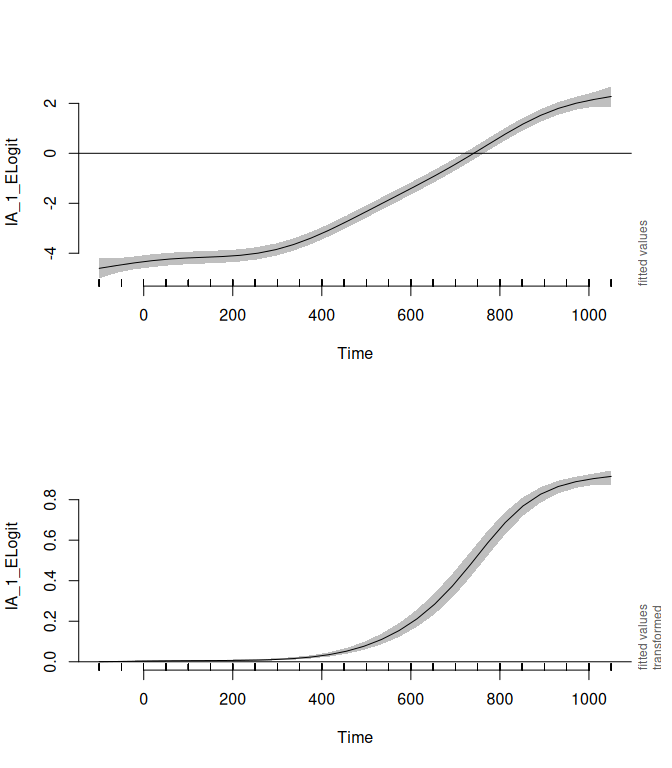The function plot_transformation_app opens a Shiny App which allows the user to visualize the effect of both number of observations and constant on the result of the empirical logit transformation and weight calculations. These values are plotted against proportions (0 to 1).
plot_transformation_app()